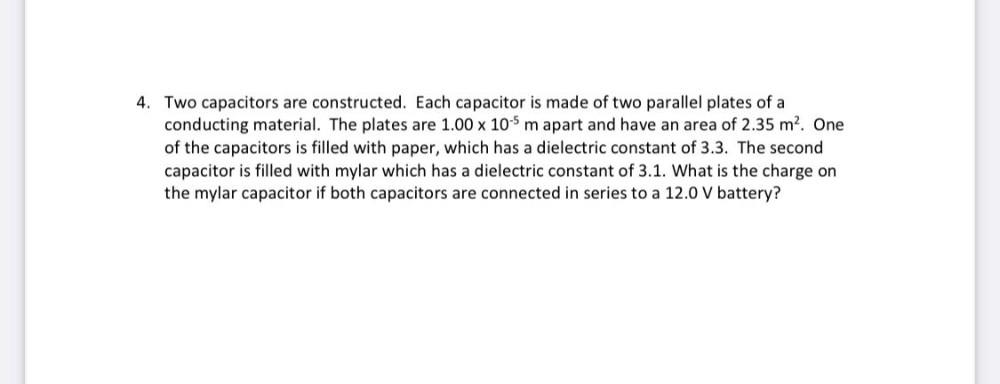Question:

# 4. Two capacitors are constructed. Each capacitor is made of two parallel plates of a conducting material. The plates are 1.00 x4. Two capacitors are constructed. Each capacitor is made of two parallel plates of a conducting material. The plates are 1.00 x 10-5 m apart and have an area of 2.35 m². One of the capacitors is filled with paper, which has a dielectric constant of 3.3. The second capacitor is filled with mylar which has a dielectric constant of 3.1. What is the charge on the mylar capacitor if both capacitors are connected in series to a 12.0 V battery?